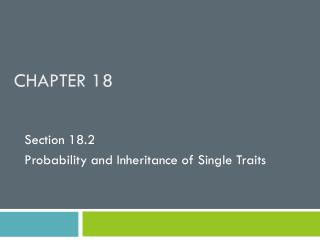DownloadDownload PresentationChapter 18

# Chapter 18

Download Presentation## Chapter 18

- - - - - - - - - - - - - - - - - - - - - - - - - - - E N D - - - - - - - - - - - - - - - - - - - - - - - - - - -
##### Presentation Transcript

1. Chapter 18 Section 18.2 Probability and Inheritance of Single Traits

2. Phenotypic Ratios • A phenotypic ratio is the ratio of offspring with the dominant trait to the recessive trait. • A phenotypic ratio can be expressed as a decimal, a fraction or a percentage. • Ex. 0.75 , ¾ or 75% are round seeded.

3. Phenotypes and Probability • The phenotypic ratio also lets us know the probability of which trait the offspring will receive. • Referring to the example from before, the offspring has a 75% chance of having round seeds and only a 25% chance of having wrinkled seeds.

4. Punnett Squares • A Punnett square is a chart used to predict the outcome of a genetic cross. • Ex. • These offspring have a 50% chance of having round seeds and a 50% chance of having wrinkled seeds.

5. Punnett Squares Cont’d • Punnett squares also allow us to determine the expected genotypic ratio. • A genotypic ratio is the ratio of offspring with each possible allele combination. • For example it allows us to see the probability of the individuals being homozygous recessive, homozygous dominant or heterozygous dominant.

6. Punnett Squares Cont’d • Ex. • This individual has a 100% chance of having round seeds. • But only has a 50% chance of being homozygous vs. heterozygous.

7. Test Crosses • A test cross is the cross of an individual of unknown genotype but known phenotype to an individual that is known, usually homozygous recessive. • The phenotypes of the F1 generation will reveal the genotype of the unknown individual.

8. Sample Test Cross • Ex. A white ram is crossed with a black sheep. White colouring is dominant over black. The black sheep must be homozygous recessive in order to be black, but the white ram can either be homozygous or heterozygous. • Crossing them and seeing the phenotypes of the offspring will reveal whether the white ram is homozygous or heterozygous.

9. Sample Test Cross Cont’d If all the offspring are white, the ram must be homozygous. If any offspring are black then the ram must be heterozygous.

10. Example Problem • A horticulture worker has seeds from a particular cross, but has no information about the genotype or the phenotype of the parents. He plants and grows the offspring, and records the traits of each offspring. What is the genotype and phenotype of the parent plants?

11. Solving the Problem • Determine the phenotypic ratio. • 5472/1850 = 2.95 which is approximately a 3/1 ratio. • Now try the different crosses to see which genotypes will give a 3/1 phenotypic ratio.

12. Solution to Example Problem 4:0 ratio 2:2 ratio Only when both parents are heterozygous will a 3:1 ratio be seen. 3:1 ratio